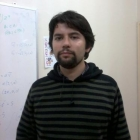﻿﻿ CI²MA - Publications | Graduate Thesis

# Graduate Thesis of Felipe LepeProgramMaster in Mathematics, Applied Mathematics, Universidad del Bío-Bío
Enrollment Year2010
Senior Year2013
Thesis TitleLocking-free finite element method for a bending moment formulation of timoshenko beams

#### Thesis Summary:

In the present thesis work the objective of the analysis of a problem of beams governed by the equations of the model of Tymoshenko, where the beam to be considered is not homogeneous in its domain. All this work will be organized as follows: In chapter two we present the three models most studied in beam theory, emphasizing the differences between these models, indicating the physical quantities involved and the respective differential equations that govern them, showing how the nature in which we can find A beam determines the boundary conditions that these differential equations will have associated. In the third chapter we will show some important results that we use in the thesis, belonging to , in particular, a theorem associated to the regularity of solution for the problem of the homogeneous beam, which shows the non dependence of the thickness of the beam, And considering as auxiliary variable only the shear force. Finally, the fourth chapter corresponds to the main result achieved in this thesis, in which the study of a non-homogeneous embedded beam is considered. We will analyze a mixed variational formulation that involves, as variables, displacement, rotation, shear stress and momentum. We will analyze the discrete problem associated with the continuum with classical finite elements, where we will see that the thickness of the beam does not depend on the convergence of this method and therefore the blocking phenomenon that usually cause this type of parameters does not affect in the calculation of error. We will show a linear convergence order in terms of mesh size, and a quadratic order in the L2 norms of the shear stress and the bending moment. We will also provide some numerical tests made with the Matlab program that verify the theoretical results obtained.

Thesis Director(s) David Mora, Rodolfo Rodríguez
Thesis Project Approval Date2012, March 14
Thesis Defense Date2013, March 30
Professional MonitoringStudent in the PhD Program in Applied Sciences with mention in Mathematical Engineering, Universidad de Concepción. From March 2013
PDF ThesisDownload Thesis PDF#### ISI Publications from the Thesis

Felipe LEPE, David MORA, Rodolfo RODRíGUEZ: Locking-free finite element method for a bending moment formulation of Timoshenko beams. Computers & Mathematics with Applications, vol. 68, 3, pp. 118-131, (2014).

<< Back to list of Graduate Thesis.

 CI²MA, CENTER FOR RESEARCH IN MATHEMATICAL ENGINEERING, UNIVERSIDAD DE CONCEPCIÓN - MAILBOX 160-C, CONCEPCIÓN, CHILE, PHONE: +56-41-2661324